# Common LaTeX Stuff for Boolean Algebra

Writing mathematical equations properly on a computer isn’t hard, and LaTeX is probably the best tool for it, and is supported by both

• M$Office; and • Apple iWork Later on when you are working in IT, Math, or Physics, LaTeX will also be the preferred tool to create PDFs in both the industry and academia. This document only introduces you to the math bits needed for Boolean algebra, but I’ve seen math students taking post-secondary math notes using LaTeX, very efficient once you are familiar with it. If you are on macOS, stick to Apple Pages, it saves you a lot of time. Simply do Insert -> Equation and you’ll be able to start writing LaTeX math without any issue, it also gives you live preview, and Apple has a pretty decent support page for it: https://support.apple.com/en-us/HT202501 Something that we recently found out though, M$ Office has the worse support for proper LaTeX grammar, so you might want to be careful there. It seems that M$takes LaTeX input and converts it (incorrectly) to its own proprietary format instead of having a real rendering engine. To setup LaTeX equation writing in M$, first select Insert -> Equation, then make sure you select LaTeX when creating it: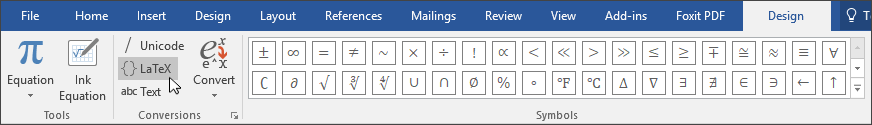Taken from M$Support ## Basic Symbols Writing LaTeX is kinda like writing code, except that this code is not to be executed but rendered. Standard symbols and numbers and letters will be rendered directly, like the plus sign and equal sign. Power is also simple with the ^ symbol e.g.: Basic stuff: y=a^2+bx+c will be rendered as: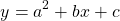Power: a^{123} will be rendered as: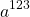Footnotes: a_{16} will be rendered as: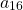Fractions: \frac{b}{a+c} will be rendered as: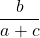A pair of curly brackets is used to indicate whatever is inside is a single component. Special symbols require you to start writing its name with right slash \ For example: \Sigma gets rendered as: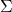\Pi gets rendered as: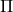\oplus gets rendered as: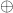\cdot gets rendered as:Make sure to also use space bar to separate different symbols. ## Overlines Overlines (NOT operators) in LaTeX is supposed to be simple. \overline{X} gets rendered as: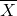\overline{X+Y} gets rendered as: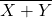\overline{X}\overline{Y} gets rendered as: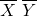For some reason that last one above might not be rendered correctly in older versions of M$ Office, you will have to do one of the below:

Use \cdot: \overline{X} \cdot \overline{Y} gets rendered as: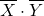Use right slash and whitespace: \overline{X} \  \overline{Y} gets rendered as: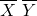Hopefully, this is enough for most boolean algebra equations. If you have more suggestions please let me know.